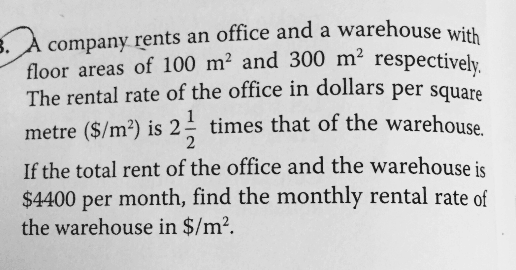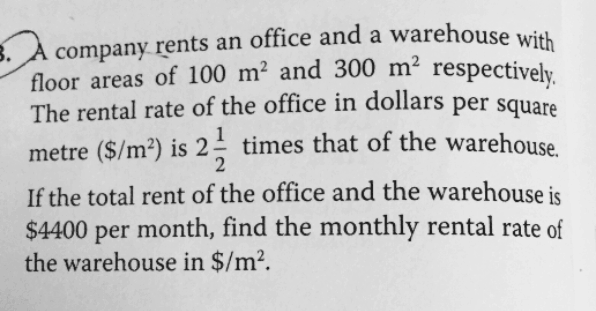# QuestionAnyone help me with attached question

Thanksthe rate of areas=1:3

the rate of price per square=2.5:1

so,

the rate of total amount=2.5:3=5:6

the amount per unit=4400/11=400

the total amount of warehouse=400*6=2400

the rate of warehouse per square=2400/300=80

0 Replies 1 Like ✔Accepted Answer

4400/(100 x 2.5 + 300) = 8 (\$/sqm for warehouse)

Ans : \$8/sq m.

0 Replies 1 Likethe rate of areas=1:3

the rate of price per square=2.5:1

so,

the rate of total amount=2.5:3=5:6

the amount per unit=4400/11=400

the total amount of warehouse=400*6=2400

the rate of warehouse per square=2400/300=80

0 Replies 1 Like